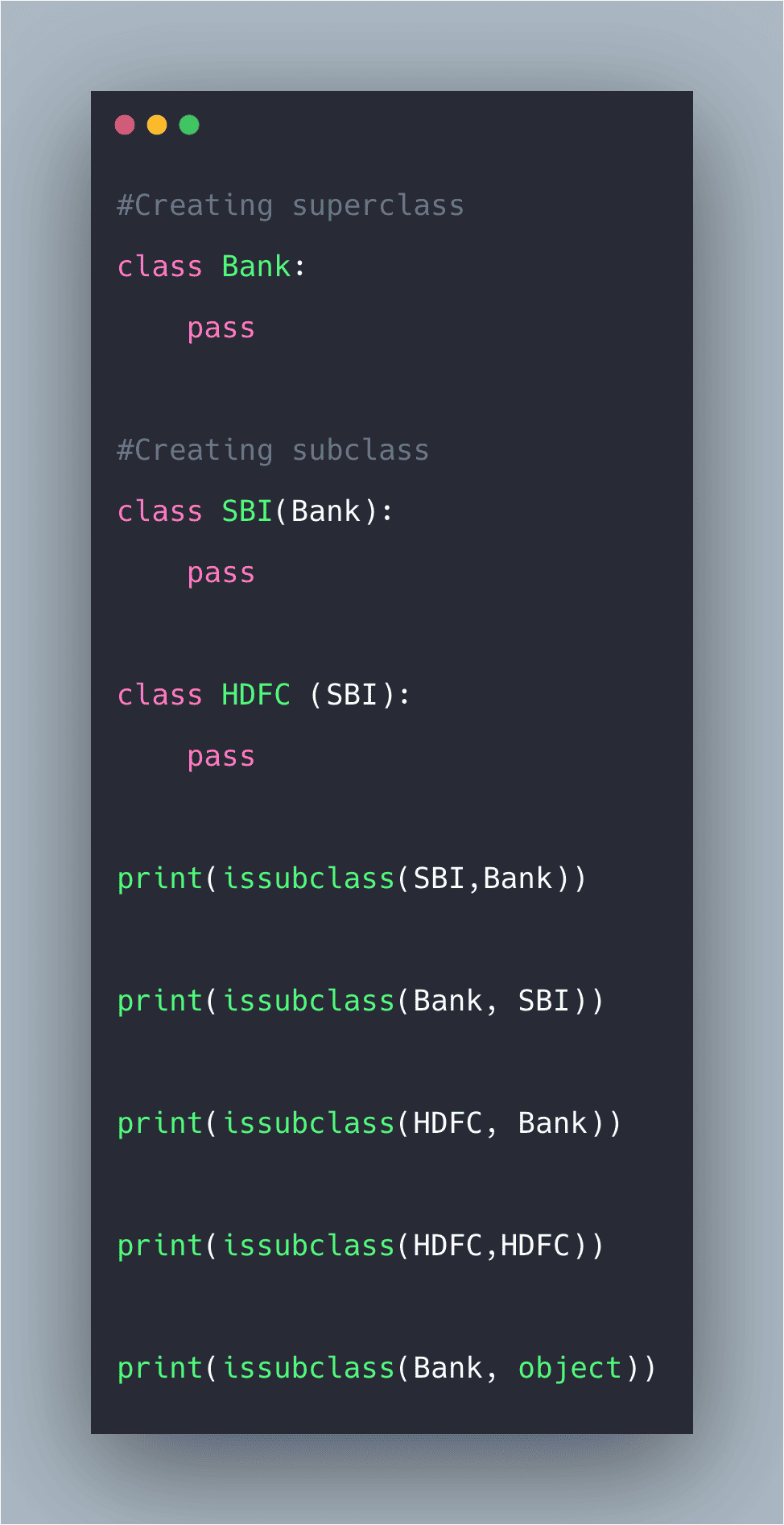# Python issubclass: How to Use issubclass() Function

1
40The issubclass() function checks if the object argument (first argument) is a subclass of classinfo class (second argument).

## Python issubclass

Python issubclass() is a built-in method that is used to check if a class is a subclass of another class or not. The issubclass() function returns boolean True if the specified object is the subclass of the specified object, otherwise False. In this method, the first argument is checked with the second argument that if the first one is the subclass of the second one or not.

### Syntax

`issubclass(object, classinfo)`

### Arguments

Here are the following parameters.

object:  is the class to be checked.

classinfo: is a class, tuple, or type of a class.

### Return value

Python issubclass() function returns two types of result, True or False.

If the object is a subclass of classinfo, then it returns True; otherwise, it returns False.

### Example

See the following code example.

```#Creating superclass
class Bank:
pass

#Creating subclass
class SBI(Bank):
pass

#Creating sub class of SBI
class HDFC (SBI):
pass

#Now we will check subclass with different arguments

#Checking if SBI is subclass of Bank or not
print(issubclass(SBI,Bank))

#Checking if Bank is subclass of SBi or not
print(issubclass(Bank, SBI))

#Checking multilevel subclass
print(issubclass(HDFC, Bank))

#Checking if a class is subclass of itself or not
print(issubclass(HDFC,HDFC))

#checking with an object argument
#Here object is referred to base class always
print(issubclass(Bank, object))```

See the output.

```True
False
True
True
True```

So, it returns True if the class supplied as the first argument is the subclass of another class supplied as the second argument, otherwise, it returns False.

## Python issubclass() with a tuple of classes

See the following code.

```# app.py

class Top:
pass

class Middle(Top):
pass

class Low(Middle):
pass

print(issubclass(Low, (str, list, Top)))
```

In the above example, you can say that Top is the main parent class, then its child class is Middle, and the Middle class’s child class is Low.

Next in the print statement, we are checking Low-class type is the subclass of a single class or tuple of classes. See the output.

```➜  pyt python3 app.py
True
➜  pyt```

## Difference between issubclass() and isinstance()

Python isinstance(object, classinfo) checks whether or not the object is an instance or subclass of classinfo.

Whereas, issubclass(class, classinfo) checks whether or not a class is a subclass of classinfo.

Python isinstance() function is used to check if the object is an instance of the specific class or any of its subclass. It takes two parameters.

The first is an object to test, and the second is a single class or a tuple of classes. It will check if the first parameter, thee object, is the instance of class classinfo.

If the second parameter is the tuple of classes, it will check if the first object is the instance of any of the classes in the tuple.

Again, the issubclass() is used to check if the class type is the subclass of a different class. The second parameter is a single class or a tuple of classes.

That’s it for this tutorial.

## Recommended Posts

Python Modules

Python dir()

Python Eval Function

Python Subprocess

Python Requests

#### 1 Comment

1.Amit Parmar

Thanks for the post

This site uses Akismet to reduce spam. Learn how your comment data is processed.